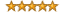# Interview Questions for Business Analysts and Systems Analysts

Recent Interview Questions | Search | Subscribe (RSS)

?
INTERVIEW QUESTION:

# What is an IF… THEN… ELSE statement?// 2681 Views // 0 Additional Answers & Comments

Categories: Analytical and Problem Solving Skills

The IF… THEN… ELSE statement is probably the most basic logical construct used to control the flow of logic.  This type of conditional statement can be found in most programming languages but it is also commonly used when expressing logic as pseudocode.

Here’s an example:

IF temperature is higher than 75 degrees THEN

Turn on the air conditioner

ELSE

Turn off the air conditioner

END-IF

The structure of an IF… THEN… ELSE statement is as follows:

IF <logical condition which can be true or false> THEN <what to do if the condition is true> ELSE <what to do if the condition is false>.

You probably noticed in the earlier example that the statement in pseudocode format has an END-IF at the end.  This is to clearly show where the list of things to do as part of the ELSE part ends.   So the IF… THEN… ELSE statement format is better written as follows (as pseudocode):

IF <logical condition which can be true or false> THEN

<what to do if the condition is true>

ELSE

<what to do if the condition is false>

END-IF

The structure of the statement can be made just slightly more complicated (and useful) by introducing the ELSE-IF construct.  It looks like this:

IF <logical condition which can be true or false> THEN

<what to do if the condition is true>

ELSE-IF <another logical condition which can be true or false> THEN

<what to do if the second condition is true>

ELSE

<what to do if the condition is false>

END-IF

The IF/THEN/ELSE statement can have as many ELSE-IF statements as needed with the assumption that the logical conditions of the IF statement and the follow-on ELSE-if statements are mutually exclusive.

Here’s the expanded example we gave above (you’ll see how useful the ELSE-IFs are):

IF temperature is higher than 75 degrees THEN

Turn on the air conditioner

ELSE-IF temperature is lower than 65 degrees THEN

Turn on the heater

ELSE

Turn off the air conditioner

Turn off the heater

END-IF

Examples of IF… THEN… ELSE statements from various programming languages

IF… THEN… ELSE in Visual Basic

If count = 0 Then

message = "There are no items."

ElseIf count = 1 Then

message = "There is 1 item."

Else

message = \$"There are {count} items."

End If

IF… THEN… ELSE in Java

if (testscore >= 90) {

} else if (testscore >= 80) {

} else if (testscore >= 70) {

} else if (testscore >= 60) {

} else {

}

IF… THEN… ELSE in Python

if b > a:

print("b is greater than a")

elif a == b:

print("a and b are equal")

else:

print("a is greater than b")

## RATE THIS TOPIC

Only registered users may post comments.

## Select ModernAnalyst Content

brought to you byenabling practitioners & organizations to achieve their goals using:

## Community Resources

Copyright 2006-2023 by Modern Analyst Media LLC